##### Print Instructions

NOTE: Only your test content will print.
To preview this test, click on the File menu and select Print Preview.

See our guide on How To Change Browser Print Settings to customize headers and footers before printing.

# Functions in Word (Ribbons and Tabs) (Grade 10)

Print Test (Only the test content will print)

## Functions in Word (Ribbons and Tabs)

1.
Explain the function of the circled item in the image below.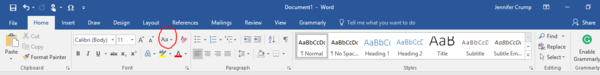2.
Explain the function of the circled item in the image below.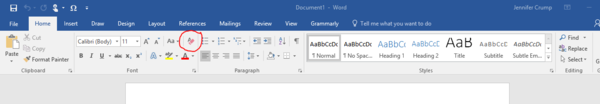3.
Explain the function of the circled item in the image below.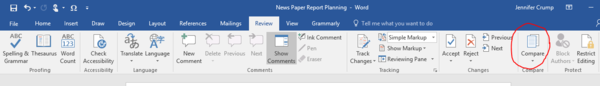4.
Explain the function of the circled item in the image below.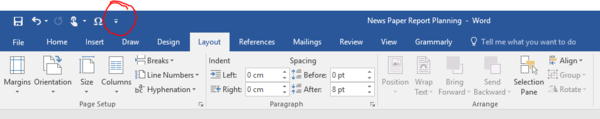5.
Explain the function of the circled item in the image below.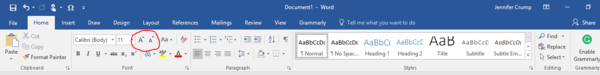6.
Explain the function of the circled item in the image below.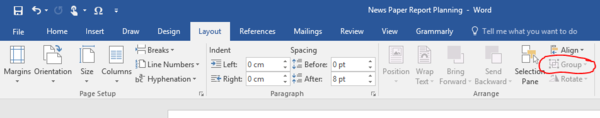7.
Explain the function of the circled item in the image below.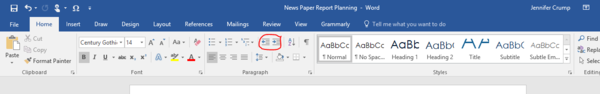8.
Explain the function of the circled item in the image below.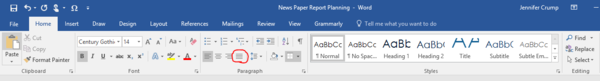9.
Explain the function of the circled item in the image below.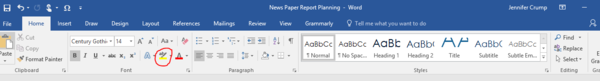10.
Explain the function of the circled item in the image below.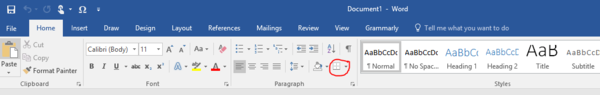11.
Explain the function of the circled item in the image below.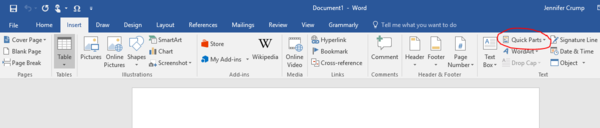12.
Explain the function of the circled item in the image below.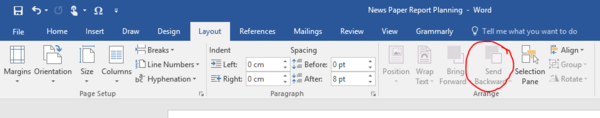13.
Explain the function of the circled item in the image below.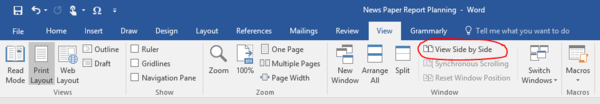14.
Explain the function of the circled item in the image below.15.
Explain the function of the circled item in the image below.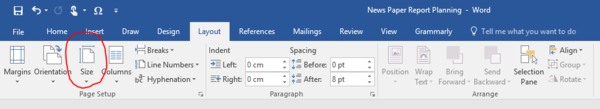16.
Explain the function of the circled item in the image below.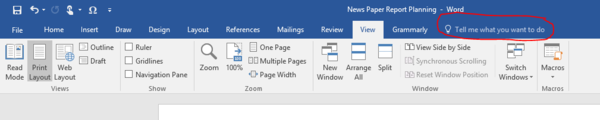17.
Explain the function of the circled item in the image below.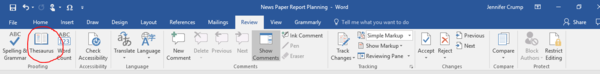18.
Explain the function of the circled item in the image below.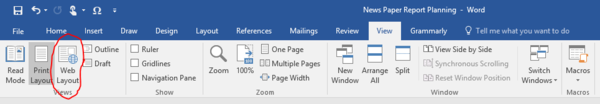19.
Explain the function of the circled item in the image below.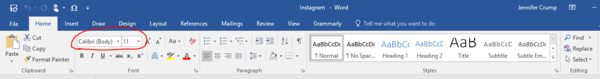20.
Explain the function of the circled item in the image below.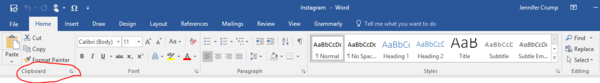You need to be a HelpTeaching.com member to access free printables.